A Source Book in Physics

Contents:
Author: James Clerk Maxwell  | Date: 1860

Show Summary
Physics

# The Distribution of Molecular Velocities

So many of the properties of matter, especially when in the gaseous form, can be deduced from the hypothesis that their minute parts are in rapid motion, the velocity increasing with the temperature, that the precise nature of this motion becomes a subject of rational curiosity. Daniel Bernoulli, Herapath, Joule, Krönig, Clausius, &c. have shown that the relations between pressure, temperature, and density in a perfect gas can be explained by supposing the particles to move with uniform velocity in straight lines, striking against the sides of the containing vessel and thus producing pressure. It is not necessary to suppose each particle to travel to any great distance in the same straight line; for the effect in producing pressure will be the same if the particles strike against each other; so that the straight line described may be very short. M. Clausius has determined the mean length of path in terms of the average distance of the particles, and the distance between the centres of two particles when collision takes place. We have at present no means of ascertaining either of these distances; but certain phaenomena, such as the internal friction of gases, the conduction of heat through a gas, and the diffusion of one gas through another, seem to indicate the possibility of determining accurately the mean length of path which a particle describes between two successive collisions. In order to lay the foundation of such investigations on strict mechanical principles, I shall demonstrate the laws of motion of an indefinite number of small, hard, and perfectly elastic spheres acting on one another only during impact.

If the properties of such a system of bodies are found to correspond to those of gases, an important physical analogy will be established, which may lead to more accurate knowledge of the properties of matter. If experiments on gases are inconsistent with the hypothesis of these propositions, then our theory, though consistent with itself, is proved to be incapable of explaining the phaenomena of gases. In either case it is necessary to follow out the consequences of the hypothesis.

Instead of saying that the particles are hard, spherical, and elastic, we may if we please say that the particles are centres of force, of which the action is insensible except at a certain small distance, when it suddenly appears as a repulsive force of very great intensity. It is evident that either assumption will lead to the same results. For the sake of avoiding the repetition of a long phrase about these repulsive forces, I shall proceed upon the assumption of perfectly elastic spherical bodies. If we suppose those aggregate molecules which move together to have a bounding surface which is not spherical, then the rotatory motion of the system will store up a certain proportion of the whole vis viva, as has been shown by Clausius, and in this way we may account for the value of the specific heat being greater than on the more simple hypothesis.

. . . . . . . . . . . . . . . . . . .

Prop. IV. To find the average number of particles whose velocities lie between given limits, after a great number of collisions among a great number of equal particles.

Let N be the whole number of particles. Let x, y, z, be the components of the velocity of each particle in three rectangular directions and let the number of particles for which x lies between x and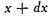be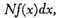where f(x) is a function of x to be determined.

The number of particles for which y lies between y and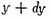will be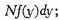and the number for which z lies between z and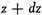will be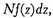where f always stands for the same function.

Now the existence of the velocity x does not in any way affect that of the velocities y or z, since these are all at right angles to each other and independent, so that the number of particles whose velocity lies between x and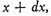and also between y and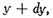and also between z and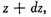is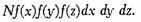If we suppose the N particles to start from the origin at the same instant, then this will be the number in the element of volume (dx dy dz) after unit of time, and the number referred to unit of volume will be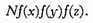But the directions of the coordinates are perfectly arbitrary, and therefore this number must depend on the distance from the origin alone, that is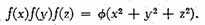Solving this functional equation, we find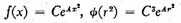If we make A positive, the number of particles will increase with the velocity, and we should find the whole number of particles infinite. We therefore make A negative and equal to −1/α2, so that the number between x and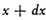is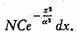Integrating from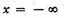to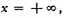we find the whole number of particles,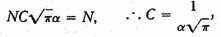f(x) is therefore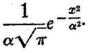Whence we may draw the following conclusions:—

1st. The number of particles whose velocity, resolved in a certain direction, lies between x and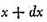is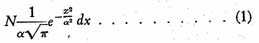2nd. The number whose actual velocity lies between v and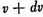is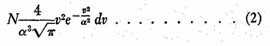3rd. To find the mean value of v, add the velocities of all the particles together and divide by the number of particles; the result is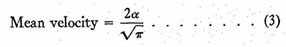4th. To find the mean value of v2, add all the values together and divide by N,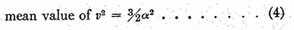This is greater than the square of the mean velocity, as it ought to be.

It appears from this proposition that the velocities are distributed among the particles according to the same law as the errors are distributed among the observations in the theory of the "method of least squares." The velocities range from 0 to ∞, but the number of those having great velocities is comparatively small. In addition to these velocities, which are in all directions equally, there may be a general motion of translation of the entire system of particles which must be compounded with the motion of the particles relatively to one another. We may call the one the motion of translation, and the other the motion of agitation.

. . . . . . . . . . . . . . . . . . .

Contents:

### Related Resources

James Clerk Maxwell

Title: A Source Book in Physics

Select an option:

## Email Options

Title: A Source Book in Physics

Select an option: